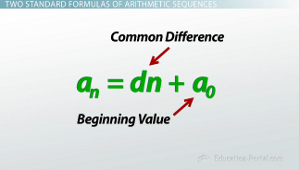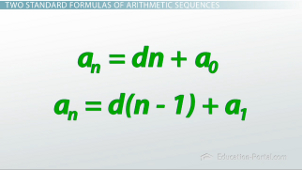# How to Find and Classify an Arithmetic Sequence

An error occurred trying to load this video.

Try refreshing the page, or contact customer support.

Coming up next: Finding and Classifying Geometric Sequences

### You're on a roll. Keep up the good work!

Replay
Your next lesson will play in 10 seconds
• 0:06 The Arithmetic…
• 2:34 Creating the Formula…
• 4:10 Two Standard Formulas…
• 6:13 Finding an Arithmetic…
• 8:33 Lesson Summary
Save Save

Want to watch this again later?

Timeline
Autoplay
Autoplay
Speed Speed

#### Recommended Lessons and Courses for You

Lesson Transcript
Instructor: Zach Pino
Arithmetic sequences are everywhere, and with a few tricks you learn here, you could end up looking like a psychic the next time you go to a movie or a football game!

## The Arithmetic Sequence of Stadium Seating

A sequence is just a pattern, and an arithmetic sequence is one that is generated through repeated addition. So each term in the sequence is created by adding the same thing to the last one, over and over again. For example, we could get the finite arithmetic sequence 11, 13, 15, 17, 19 simply by starting with 11 and adding 2 four times. Or maybe we could start with 30 and repeatedly add -10 to get the infinite arithmetic sequence 30, 20, 10, 0, -10… and on and on and on.

Now as it turns out, we actually see these patterns in a lot of places. Take an auditorium for example; they can't fit many seats in the front row because the stage is pretty small, but as you go further back, each row of seating has more and more seats because there is more space. Let's imagine a theater where there are 35 seats in the front row, and 4 more seats in every row behind it. That would make 39 in the second row, 43 in the third row, 47 in the fourth row, 51 in the fifth row and so on.Describing this sequence in words is good enough for now, and can help us answer questions like, how many seats are in the 10th row? Well okay, lets see, if we can just continue the pattern, that would mean that there are 55 in the 6th, 59 in the 7th, then 63 in the 8th, 67... finally, 71 seats in the 10th row.

But if it turns out that we're in the University of Michigan Stadium, which has close to 100 rows of seats, would the words and pattern really help us figure out how many seats were in the 91st row? Not so much. Not only would this be incredibly tedious, but there's also a good chance we'd end up making a small mistake and our answer would be off by a few seats.

What we really need is a formula that we can just plug 91 into and it'll spit out exactly how many seats are in that row for us. The way we can do that is by noticing that an arithmetic sequence is really a linear relationship. Our current example, 35, 39, 43, 47… this is linear because it is increasing by the same amount each step, in this case, 4.

## Creating the Formula of an Arithmetic Sequence

If we were going to write this as a linear equation, we'd probably end up using slope-intercept form: y=mx+b. Remember that m tells us the slope, or how much it moves by, and b is the y-intercept, or where it begins when x is equal to 0.

These two things are fairly straightforward to find in our pattern; the slope in this case (how much it's moving by) is simply 4. We keep on adding 4 each time so in our equation where we see m, we put a 4. The b (where it begins or the y-intercept), is a little more complicated because you have to realize that this is where x equals 0, and our first term is exactly that - the first term, a1. We need a0, which means that we do have to go backwards one entry; to go backwards we don't add 4, but we subtract 4. It looks like if there was one row closer it would have 31 seats, which means my b value - my y-intercept - is 31. I get the equation y = 4x+31.Now because we're treating this as a sequence, while our rule will stay the same, we're going to use different letters to write the rule. In the place of y, we're going to put a subscript n, which will stand for the nth term, and in place of x we'll put n. So if I want the twelfth term, I can plug 12 in for n.

## Two Standard Formulas of Arithmetic Sequences

This brings us the rule for the nth row of our stadium as an = 4n+31. All arithmetic rules will look something like this and they will be an = dn+a0. The a0 obviously represents the beginning value, kind of like the b or the y-intercept, and d(what used to be called the slope) is now going to be called the common difference because each term in our sequence has a common difference between them.

To unlock this lesson you must be a Study.com Member.

### Register to view this lesson

Are you a student or a teacher?

#### See for yourself why 30 million people use Study.com

##### Become a Study.com member and start learning now.
Back
What teachers are saying about Study.com

### Earning College Credit

Did you know… We have over 200 college courses that prepare you to earn credit by exam that is accepted by over 1,500 colleges and universities. You can test out of the first two years of college and save thousands off your degree. Anyone can earn credit-by-exam regardless of age or education level.